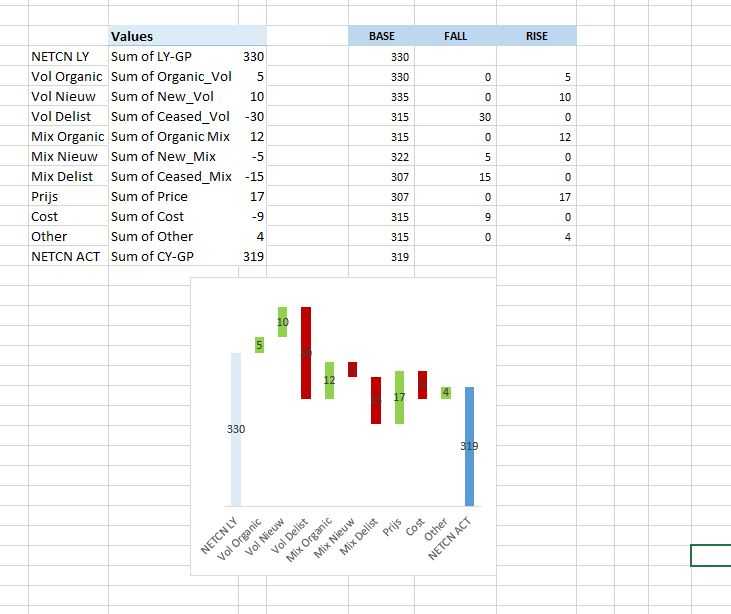# New to QlikView

Discussion board where members can get started with QlikView.

Announcements
Leverage your QlikView investment to modernize BI – see how! Join Group
cancel
Showing results for
Did you mean:
HighlightedContributor III

## Sumif function

Hi Guys!

Just trying to figure out the sumif equivalent in Qlikview.

From the forum I understood, it's =sum(if(.....

However I'm having some difficulties. So my aim is to get the total Net Contribution (NETCN) per account group.

I've followed all the steps on the forum, but for some reason the formula isn't working. Anyone has an idea?

=sum(if([Account Group], sum({<GL_1={NETCN},Dimension={ACT}>}Data)))

10 Replies
HighlightedMVP

## Re: Sumif function

Take a straight table:

Dimension: [Account Group]

Exp: sum({<GL_1={NETCN},Dimension={ACT}>}Data)

If this is not what you want. Try to share a sample data and explain expected output against that.

HighlightedContributor III

## Re: Sumif function

Ah sorry, I'm trying to set up a waterfall so it needs to be in a graph. After figuring out the sumif function in Qlikview I'll add another sumif behind it, getting a standard rate per bottle sold

=sum(if([Account Group], sum({<GL_1={NETCN},Dimension={ACT}>}Data)))  /

sum(if([Account Group], sum({<GL_1={VOL},Dimension={ACT}>}Data)))

HighlightedMVP

## Re: Sumif function

What is your dimension in the chart? Could share your sample app?

HighlightedSpecialist III

## Re: Sumif function

Have you tried using:

Sum({<GL_1={'NETCN'},Dimension={'ACT'}>}Data) / Sum({<GL_1={'VOL'},Dimension={'ACT'}>}Data)

HighlightedContributor III

## Re: Sumif functionI have nothing yet, I'm trying to set up something similar as above

HighlightedCreator

## Re: Sumif function

Hi

I think its best to deal it in the Script

sumif in scripts

HighlightedMVP

## Re: Sumif function

Try to share the excel file so that we can work on it to help you.

HighlightedContributor III

## Re: Sumif function

Trying to send an excel file here, but doesn't work... ill try to see if I can add the sumif formula in the script

HighlightedPartner

## Re: Sumif function

Hi,

if you are trying to use "sum(if(" then the syntax is this: sum(if(condition,then, else))

in our case that syntax will be: sum(if(  GL_1='NETCN' and DIMENSION='ACT', Data))

if the if-clause is true then it will give as result the values from the field "Data" and then it will be sum(Data).

the other examples you have been given already are set analysis

Hope it helps,

Andrew